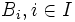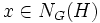# MWNSCDIN-subgroup

BEWARE! This term is nonstandard and is being used locally within the wiki. [SHOW MORE]
This article defines a subgroup property: a property that can be evaluated to true/false given a group and a subgroup thereof, invariant under subgroup equivalence. View a complete list of subgroup properties[SHOW MORE]

## Definition

### Symbol-free definition

A subgroup of a group is termed a MWNSCDIN-subgroup if it is a multiple weak normal subset-conjugacy-determined subgroup inside its normalizer relative to the whole group.

### Definition with symbols

A subgroup$H$ of a group$G$ is termed a MWNSCDIN-subgroup if, given a collection of normal subsets$A_i, i \in I$ and$B_i, i \in I$ of$H$, and an element$g \in G$ such that$gA_ig^{-1} = B_i$ for all$i \in I$, there exists$x \in N_G(H)$ such that$xA_ix^{-1} = B_i$ for all$i \in I$.